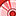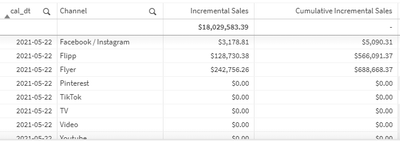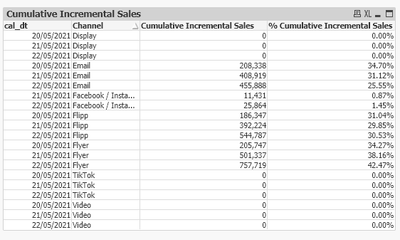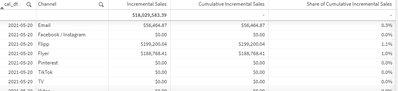Announcements
Sept. 27, 10AM ET Do more with Qlik, Insight Advisor – our intelligent AI-assistant in Qlik Sense: Register
cancel
Showing results for
Did you mean:Contributor III

## Share of Cumulative Total by Dimension

Hi,

I am trying to create an additional measure that shows the % share of Cumulative Incremental Sales by Channel and cal_dt. Right now my Incremental Sales and Cumulative Incremental Sales work as expected. The formla for Cumulative Incremental Sales is below:

Aggr(RangeSum(Above(Sum({<type-={'Control'}>}sales), 0, RowNo())),channel, (cal_dt, (NumericCount)))

How do I create a measure that will show the % of Cumulative Incremental Sales for each channel (compared to total) for every cal_dt?1 Solution

Accepted SolutionsMVP

@mpalha  create a table with below Dimensions and measure

Dimensions:

1) cal_dt

2) Channel

Measures:

1) Cumulative Incremental Sales

``=sum(aggr(rangesum(above(sum({<cal_dt,Channel>}[Incremental Sales]),0,RowNo(total))),Channel, (cal_dt,(NUMERIC,ASCENDING))))*avg(1)``

2) %Cumulative Incremental Sales

``````=(sum(aggr(rangesum(above(sum({<cal_dt,Channel>}[Incremental Sales]),0,RowNo(total))),Channel, (cal_dt,(NUMERIC,ASCENDING))))/

sum(total <cal_dt>aggr(rangesum(above(sum({<cal_dt,Channel>}[Incremental Sales]),0,RowNo(total))),(cal_dt,(NUMERIC,ASCENDING)))))*avg(1)``````10 RepliesMVP

Hi, you can try with:

Aggr(RangeSum(Above(Sum({<type-={'Control'}>}sales), 0, RowNo())),channel, (cal_dt, (NumericCount)))/ Sum(TOTAL {<type-={'Control'}>}sales)Contributor III
Author

Hi @rubenmarin ,

Thanks for the suggestion. I believe the formula is working for all dates, however the share should be split for a single cal_dt. For example, for cal_dt 2021-05-20, the share of Cumulative Incremental Sales should add up to 100% for that date. Right now this only happens when a single date is filtered in the report. Any suggestions?MVP

try below

Aggr(RangeSum(Above(Sum({<type-={'Control'}>}sales), 0, RowNo())),channel, (cal_dt, (NumericCount)))/ Sum(TOTAL <cal_dt> {<type-={'Control'}>}sales)Contributor III
Author

Thanks @Kushal_Chawda for the suggestion. The formula will only work for the first cal_dt... the share % gives values >100% for future dates.

For every date, Cumulative Incremental Sales should be totaled for all channels. Share of Cumulative Incremental Sales should display the share % (compared to that total) of Cumulative Incremental Sales for that channel for that day.MVP

Would you be able to share sample data with expected output?Contributor III
Author

Hey @Kushal_Chawda ,

Sorry for the late reply. I have attached what you asked. The expected output is the blue column. Thanks!MVP

@mpalha  how % is calculated?Contributor III
Author

Cumulative Incremental Sales is totaled for a distinct cal_dt. Then the Cumulative Incremental Sales for a Channel is divided by this total.

For example, for cal_dt 2021-05-21, total Cumulative Incremental Sales is \$1,313,911.54. For the E-mail channel, its Share of Cumulative Incremental Sales is \$408,918.58 / \$1,313,911.54 = 31%. Similarly for the Flyer channel, its Share of Cumulative Incremental Sales is \$501,337.39 / \$1,313,911.54 = 38%.

I hope this helps.MVP

@mpalha  create a table with below Dimensions and measure

Dimensions:

1) cal_dt

2) Channel

Measures:

1) Cumulative Incremental Sales

``=sum(aggr(rangesum(above(sum({<cal_dt,Channel>}[Incremental Sales]),0,RowNo(total))),Channel, (cal_dt,(NUMERIC,ASCENDING))))*avg(1)``

2) %Cumulative Incremental Sales

``````=(sum(aggr(rangesum(above(sum({<cal_dt,Channel>}[Incremental Sales]),0,RowNo(total))),Channel, (cal_dt,(NUMERIC,ASCENDING))))/

sum(total <cal_dt>aggr(rangesum(above(sum({<cal_dt,Channel>}[Incremental Sales]),0,RowNo(total))),(cal_dt,(NUMERIC,ASCENDING)))))*avg(1)``````Tags
Community Browser